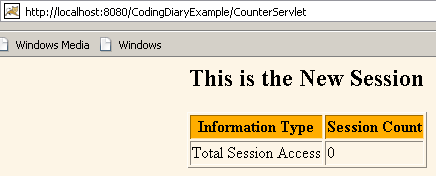Ads

Tutorials

# Hit Counter Servlet Example

This example illustrates about counting how many times the servlet is accessed.

This example illustrates about counting how many times the servlet is accessed.

# Hit Counter Servlet Example

This example illustrates about counting how many times the servlet is accessed. When first time servlet (CounterServlet) runs then session is created and value of the counter will be zero and after again accessing of servlet  the counter value will be increased by one. In this program isNew() method is used whether session is new or old and getValue() method is used to get the value of counter.

Here is the source code of CounterServlet.java:

 ``` import java.io.*; import javax.servlet.*; import javax.servlet.http.*; public class CounterServlet extends HttpServlet{   public void doGet(HttpServletRequest request,    HttpServletResponse response)   throws ServletException, IOException {   HttpSession session = request.getSession(true);   response.setContentType("text/html");   PrintWriter out = response.getWriter();   Integer count = new Integer(0);   String head;   if (session.isNew()) {   head = "This is the New Session";   } else {   head = "This is the old Session";   Integer oldcount =(Integer)session.getValue("count");    if (oldcount != null) {   count = new Integer(oldcount.intValue() + 1);   }   }   session.putValue("count", count);   out.println("\n" +   "" + head + "\n" +    "\n"   + "\n"    +"
Information TypeSession Count\n"    +"
Total Session Accesses\n" +   "" + count + "\n" +   "\n"    +"" );   } }```
\n" +"

Mapping of Servlet ("CounterServlet.java") in web.xml file

   CounterServlet   CounterServlet     CounterServlet   /CounterServlet

Running the servlet by this url: http://localhost:8080/CodingDiaryExample/CounterServlet  displays the figure below:When servlet is hit two times by the user the counter value will be increased by two as shown in figure below:Download Source Code

Ads

Ads